Next: The Circuit Up: Inductance Previous: Energy Stored in an

The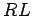Circuit

Consider a circuit in which a battery of emfis connected in series with an inductor of inductance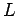and a resistor of resistance. For obvious reasons, this type of circuit is usually called ancircuit. The resistanceincludes the resistance of the wire loops of the inductor, in addition to any other resistances in the circuit.

In steady-state, the current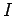flowing around the the circuit has the magnitude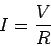(254)

specified by Ohm's law. Note that, in a steady-state, or DC, circuit, zero back-emf is generated by the inductor, according to Eq. (243), so the inductor effectively disappears from the circuit. In fact, inductors have no effect whatsoever in DC circuits. They just act like pieces of conducting wire.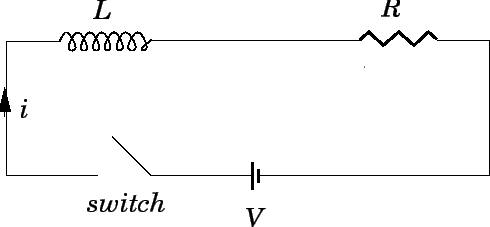Let us now slightly modify ourcircuit by introducing a switch. The new circuit is shown in Fig. 46. Suppose that the switch is initially open, but is suddenly closed at. Obviously, we expect the instantaneous currentwhich flows around the circuit, once the switch is thrown, to eventually settle down to the steady-state value. But, how long does this process take? Note that as the current flowing around the circuit is building up to its final value, a non-zero back-emf is generated in the inductor, according to Eq. (243). Thus, although the inductor does not affect the final steady-state value of the current flowing around the circuit, it certainly does affect how long after the switch is closed it takes for this final current to be established.

If the instantaneous currentflowing around the circuit changes by an amount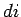in a short time interval, then the emf generated in the inductor is given by [see Eq. (243)]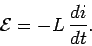(255)

Applying Ohm's law around the circuit, we obtain(256)

which yields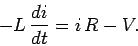(257)

Let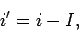(258)

whereis the steady-state current. Equation (257) can be rewritten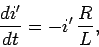(259)

since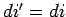(becauseis non-time-varying). At, just after the switch is closed, we expect the currentflowing around the circuit to be zero. It follows from Eq. (258) that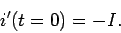(260)

Integration of Eq. (259), subject to the initial condition (260), yields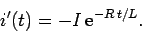(261)

Thus, it follows from Eq. (258) that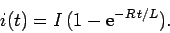(262)

The above expression specifies the currentflowing around the circuit a time intervalafter the switch is closed (at time). The variation of the current with time is sketched in Fig. 47. It can be seen that when the switch is closed the currentflowing in the circuit does not suddenly jump up to its final value,. Instead, the current increases smoothly from zero, and gradually asymptotes to its final value. The current has risen to approximately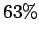of its final value a time(263)

after the switch is closed (since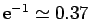). By the time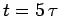, the current has risen to more than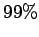of its final value (since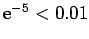). Thus,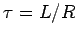is a good measure of how long after the switch is closed it takes for the current flowing in the circuit to attain its steady-state value. The quantity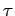is termed the time-constant, or, somewhat unimaginatively, the L over R time, of the circuit.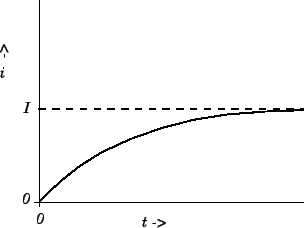Suppose that the current flowing in the circuit discussed above has settled down to its steady-state value. Consider what would happen if we were to suddenly (at, say) switch the battery out of the circuit, and replace it by a conducting wire. Obviously, we would expect the current to eventually decay away to zero, since there is no longer a steady emf in the circuit to maintain a steady current. But, how long does this process take?

Applying Ohm's law around the circuit, in the absence of the battery, we obtain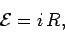(264)

where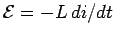is the back-emf generated by the inductor, andis the instantaneous current flowing around the circuit. The above equation reduces to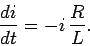(265)

At, immediately after the battery is switched out of the circuit, we expect the currentflowing around the circuit to equal its steady-state value, so that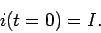(266)

Integration of Eq. (265), subject to the boundary condition (266), yields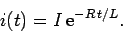(267)

According to the above formula, once the battery is switched out of the circuit, the current decays smoothly to zero. After one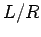time (i.e.,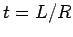), the current has decayed to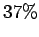of its initial value. After fivetimes, the current has decayed to less than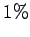of its initial value.

We can now appreciate the significance of self inductance. The back-emf generated in an inductor, as the current flowing through it tries to change, effectively prevents the current from rising (or falling) much faster than the L/R time of the circuit. This effect is sometimes advantageous, but is often a great nuisance. All circuits possess some self inductance, as well as some resistance, so all have a finitetime. This means that when we power up a DC circuit, the current does not jump up instantaneously to its steady-state value. Instead, the rise is spread out over thetime of the circuit. This is a good thing. If the current were to rise instantaneously then extremely large inductive electric fields would be generated by the sudden jump in the magnetic field, leading, inevitably, to breakdown and electric arcing. So, if there were no such thing as self inductance then every time we switched a DC electric circuit on or off there would be a big blue flash due to arcing between conductors. Self inductance can also be a bad thing. Suppose that we possess a fancy power supply, and wish to use it to send an electric signal down a wire. Of course, the wire will possess both resistance and inductance, and will, therefore, have some characteristictime. Suppose that we try to send a square-wave signal down the wire. Since the current in the wire cannot rise or fall faster than thetime, the leading and trailing edges of the signal get smoothed out over antime. The typical difference between the signal fed into the wire (upper trace) and that which comes out of the other end (lower trace) is illustrated in Fig. 48. Clearly, there is little point in us having a fancy power supply unless we also possess a low inductance wire, so that the signal from the power supply can be transmitted to some load device without serious distortion.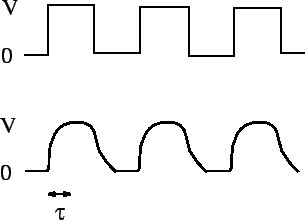Next: The Circuit Up: Inductance Previous: Energy Stored in an
Richard Fitzpatrick 2007-07-14